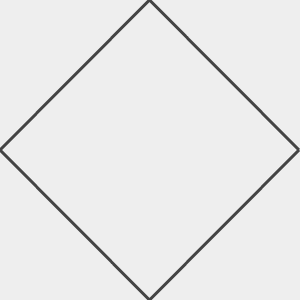# Programming Challenge #2 - Drawing

Now that we know how to count, let's try drawing, using our newly discovered counting abilities! To draw, we are first going to learn about an 'array'. An array is a list of slots where each slot can hold a value (much like a variable). You can kind of think of an array as a list of variables.

To use arrays, you use square backets `[` and `]`. You can use or change the value of slot in the array by telling the computer which 'index' you want to use. An index is a number inside the `[` and `]` and arrays always start at index `0`. For example, `array` tells the computer you want to use the first slot in the array. `array` is the second slot and `array` is the third slot.

Here's a simple example showing how we can make a new array and index into it.

```    var myArray = [];
myArray = 100;
myArray = 200;
print(myArray);
print(myArray);
```

Let's copy that example code and run it to see what happens.

## Result

Let's try to understand each piece of this code. Just like before, the `var` tells the computer that we want to have a new variable, and this time the variable is called `myArray`. We using `=` to set the variable to `[]`, which is an empty array. Just like before `;` tells the computer that we at the end of the line.

```    var myArray = [];
myArray = 100;
myArray = 200;
print(myArray);
print(myArray);
```

Now we are setting the first slot in the array. We do this by telling the computer that we are using the varible `myArray` we created above. Then we tell it we want the first slot `` (remember 0 is the first slot, 1 is the second, 2 is the third, etc.).

```    var myArray = [];
myArray = 100;
myArray = 200;
print(myArray);
print(myArray);
```

And just like before, we are using `=` to set a value. This time we are setting the first slot of `myArray` using index ``, and we are setting it to the number `100`. Again, `;` is the end of the line.

```    var myArray = [];
myArray = 100;
myArray = 200;
print(myArray);
print(myArray);
```

The next line is very simliar to the previous one, but now we are setting the second slot of the array by using index `` and we setting it to the number `200`.

```    var myArray = [];
myArray = 100;
myArray = 200;
print(myArray);
print(myArray);
```

The next two lines use that `print` function to display the values in the array. `myArray` is the first slot and `myArray` is the second slot.

```    var myArray = [];
myArray = 100;
myArray = 200;
print(myArray);
print(myArray);
```

Now let's try to combine what we learned in the first challenge with an array. Let's fill up an array with the numbers from 0 to 10,000 counting by 100's. We use `*` for multiplication and in this case, we are multiplying by `100`.

```    var myArray = [];
for (var i = 0; i < 101; i = i + 1) {
myArray[i] = i * 100;
}
print(myArray);
```

Let's copy that example code and run it to see what happens.

## Result

Hopefully you understand how and why that works. If not, ask Mommy or Daddy.

Here's another way to fill up an array.

```    var myArray = [];
for (var i = 0; i < 101; i = i + 1) {
myArray.push(i * 100);
}
print(myArray);
```

You may have noticed a new `push` function that we used here. It pushes a new number onto the end of the array. For example, if your array has the numbers `5` and `8` in it `[ 5, 8 ]` and then you `push(10)` on it, you'll get an array of `[ 5, 8, 10 ]`.

Let's copy that example code and run it to see what happens.

# Finally We Get to Draw

Now for something a little different. Let's try drawing using an array. We are going to draw in a box where the number `0` is the middle of the box, `-150` is the bottom of the box, and `150` is the top of the box. Each value in the array will get drawn in the box from left to right.

This might be easier to understand with an example

If you wanted to draw something across the middle of the box, you could do this:

```    var myArray = [];
for (var i = 0; i < 151; i = i + 1) {
myArray.push(0);
}
draw(myArray);
```

Give it a try! You can try changing `push(0)` to `push(150)` to make it go across the top. Or `push(-150)` to go across the bottom.

## Result

Now let's make it a little more interesting.

```    var myArray = [];
for (var i = 0; i < 151; i = i + 1) {
myArray.push(i);
}
draw(myArray);
```

This will draw a line from the middle of the box to the top-right corner. Try it!

# The First Challenge

Write code that draws a line from the middle of the box to the bottom-right corner.

# The Second Challenge

Write code that draws a line from the bottom-left of the box to the top-right corner.

# The Third Challenge

Write code that draws an X across the box. It should look like this:You might need two arrays for this one!

# The Super Challenge

Write code that draws a diamond in the box!!!You might need 2 arrays for this one and change the direction of your for-loop in the middle of each one.